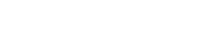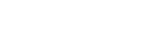# AI Introduction using Python

Romano Marinić, Junior Software Developer
03.03.2023.The subject of artificial intelligence (AI) is expanding quickly and has the potential to revolutionize a wide range of sectors. Artificial intelligence (AI) is a computer simulation of cognitive functions such as learning, reasoning, and self-correction. It combines several technologies, including robotics, computer vision, machine learning and natural language processing.

Due to its simplicity, usability and vast developer community, Python is a well-liked programming language used for AI development. TensorFlow, PyTorch and scikit-learn are just some of the tools and frameworks available in Python that facilitate the development of AI applications.

In this post, we'll introduce you to Python-based artificial intelligence (AI) and provide you a basic example of how to apply AI with code snippets.

### Example: Image Recognition

A computer's ability to recognize objects, people, buildings, and other items in an image is known as image recognition. The TensorFlow library is used in the following code to create a basic image recognition model.

TensorFlow, a well-known deep learning package for Python, is used to build an image recognition model. The most popular machine learning platform, TensorFlow, is utilized by millions of developers. On GitHub, it has received the third-most stars (after Vue and React), and on PyPI, it is the most-downloaded machine learning package.

Here is a detailed breakdown of the code:

Tensorflow and Keras libraries must be imported. A high-level TensorFlow API for creating and training deep learning models is called Keras.

Load the MNIST dataset: this dataset consists of 28x28 grayscale images of handwritten digits, along with their corresponding labels. The dataset is loaded using the load_data() function from keras.datasets.mnist.

Data preparation: To normalize the pixel values to a range between 0 and 1, the data is split by 255. Since neural networks function best when given input data that has a standard distribution, this phase is crucial.

Create the model: keras.Sequential is used to create a straightforward neural network model. Three layers make up the model:

The 28x28 picture arrays are input into the first layer, a flatten layer, which converts them into one-dimensional arrays.
The model is given nonlinearity by the second layer, a 128-neuron dense layer with a ReLU activation function.

ReLU (Rectified Linear Unit) activation function: This is a popular activation function used in neural networks. It takes the input and returns the input if it is positive, and returns 0 if it is negative. This helps the model avoid saturation in the negative range and results in faster training.

The output of the third layer, a dense layer of 10 neurons with a softmax activation function, is the class probabilities for the 10 digits (0 to 9).
Compile the model: The optimizer, loss function and evaluation metrics are specified in the build method, which is used to compile the model. The evaluation metric is accuracy, the loss function is sparse categorical crossentropy, and the optimizer is adam.

Adam Optimizer: A gradient-based optimization approach called Adam (Adaptive Moment Estimation) is used to update the model's parameters as it is being trained. It is a well-liked option due to its processing efficiency and suitability for big datasets.

Sparse Categorical Crossentropy Loss Function: This loss function, known as the sparse categorical crossentropy loss function, is applied in multi-class classification issues when each input is a member of one or more classes. The 10 classes in the MNIST dataset are represented by the digits 0 through 9. The "sparse" part of the name means that the labels are integer-encoded rather than one-hot encoded. The loss function assesses the discrepancy between the true class labels and the predicted class probabilities, and it is used to control model optimization.

Model training: The model is trained using the fit approach which runs the model for 10 iterations on the training set of data.

Evaluate the model: The evaluate method is used to evaluate the model on the test data, returning the accuracy and loss for the data.

Finally, the console prints the test accuracy. With this simple model, we can achieve a test accuracy of about 98%

### Conclusion

Due to its simplicity and sizable developer community, Python is a popular choice for developing AI, which is a fast-expanding topic with enormous promise. In this article, I have presented AI using Python and gave a straightforward example of how to apply AI using code snippets. I hope that this post has given you a taste of what AI can accomplish and how simple Python is to learn.